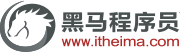# Java程序异常处理之try…catch和finally[黑马程序员]

try{

//程序代码块

} catch(ExceptionType (Exception 类及其子类) e) {

//对 ExceptionType的处理

}public class Example{

public static void main(String [] args){

//下面的代码定义了一个 try catch语句用于捕获异常

try{

int result= divide(4,0);    //调用 divide()方法

System.out.println(result);

} catch (Exception e) {     //对异常进行处理

System.out.println("捕获的异常信息为:"+e.getMessage());

}

System.out.printIn("程序继续向下执行……”);

}

//下面的方法实现了两个整数相除

public static divide (int x,int y) {

int result=X / y;    //定义一个变量result记录两个数相乘的结果

return result;      //将结果返回

}

}

D:\cn\itcast\chapter04>java Example

public class Example{

public static void main (String[] args) {

//下面的代码定义了一个try… catch…fina1ly语句用于获异常

try {

int result= divide(4，0);  //调用 divide()方法

System.out.println(result);

} catch (Exception e){        //对捕获到的异常进行处理

System.out.println(“捕获的异常信息为:”+e.getMessage());

return;     //用于结束当前语句

} finally {

System.out.println("进人finally代码块”);

}

System.out.println("程序继续向下执行……”);

}

//下面的方法实现了两个整数相除

public static int divide (int x, int y){

int result=x / y; //定义一个变量result记录两个数相除的结果

return result;   //将结果返回

}

}

D:\cn\itcast\chapter04>java Example